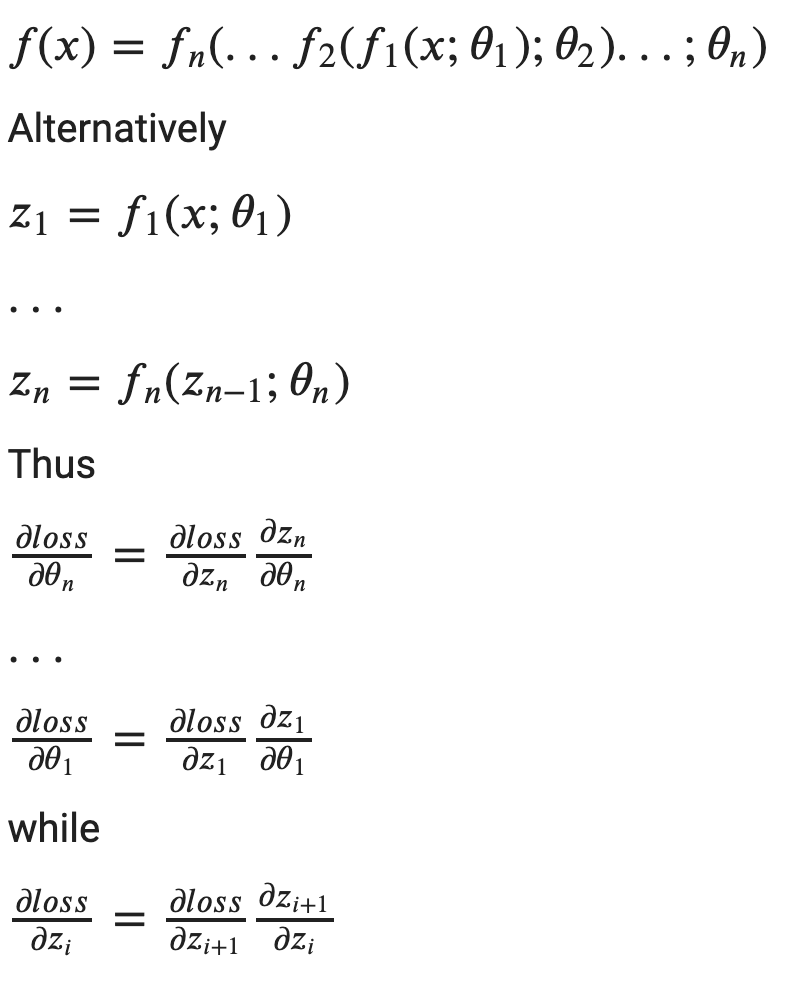# Deep Learning from scratch

Source: Deep Learning on Medium# Deep Learning from scratch

This is a quick tutorial to show you all the underlining mystery of a DL framework. It introduces basic theory that you can further extend to make a real use case while not getting too much details into the software engineering complexity of how the DL framework is implemented.

Although the goal is to do something end to end without external dependencies, we will use numpy as the tensor computation library. An introduction on how to implement numpy from scratch could be another good topic but that’s not the focus of this post.

# A bit theorm that you can skip

Deep learning is nothing but a series of complex tensor operations. Tensor is a linear algebra object that holds numeric values. Tensor could have different dimensions. Scalar is Tensor with 0 dimension, and Vector has dimension 1, while Matrix has dimension 2. Tensor could have different data type (dtype in some framworks), such as float, int, etc. All elements in the Tensor are having the same data type. Different data type has different precisions. In gerneral, as precisions goes up, the required computation power goes with it.

Deep learning network could be thought of as a numeric function, which takes in an input Tensor (often called x) and returns another Tensor (often called prediction or y). A DL network is defined by its instrinstic properties: the network structure and the weights.

When the network structure is given, training a deep learning network means simply finding the network weights for a criterion. AutoML will be the tool to look for if the network structure also needs to be found. We will not cover AutoML here.

If the criterion can be expressed numerically, DL training is to find the weights to minimize or maximize the criterion.

In this example, we want our neural network to do some linear regression and want to minimize the different between the predicted results and the golden results (often called label).

More formally, given 𝑓(𝑥;𝜃) where f is the network structure, x is the input to the model and 𝜃 represents the model weights, we want to find the best 𝜃 so that 𝑙𝑜𝑠𝑠(𝑦,𝑦̂ ) is minimized where y is the predicted result and 𝑦̂ is the label (expected outcome) for giving x.

Note that in this tutorial we limit our scope so the loss function only returns a scalar, but in multi-task learning, the loss function could have higher dimensions or there could be multiple loss functions.

The most common way of training a deep learning network is using gradient descent. Consider a multi-variable function 𝑓, the gradient of 𝑓 at a point 𝑥 (i.e. ∇𝑓(𝑥)) is a vector showing the direction in which the function rises most quickly.

A tiny movement towards the opposite direction will cause a decrease, i.e.

𝑓(𝑥−𝜆∇𝑓(𝑥))<𝑓(𝑥)

The value 𝜆 (often called 𝑙𝑟, learning rate) is a positive scalar and is chosen so that 𝑓 is monotonic between 𝑥 and 𝑥−𝜆∇𝑓(𝑥)

For a single variable function, the can be think of as finding a side which causes 𝑓(𝑥) to decrease.

In our case, we’re interested in finding 𝜃 A simple optimization strategy (simple version of SGD) is to make

𝜃=𝜃−𝑙𝑟∗∇𝜃𝑓(𝑥)

Adam is another optimization strategy that worth looking into.

To make it easy to type, let’s use w for 𝜃 and dw for ∇𝜃𝑙𝑜𝑠𝑠

`for x, y_label in dataset: y_pred = f(x, w) loss = criterion(y_label, y_pred) w -= lr * dw`

Note that we often do computation in batches, so

`x.shape = (batch, input_features)y_{label,pred}.shape = (batch, output_features)`

# Chain rule

Let’s say the network we’re working on is a sequential network, which means that 𝑓 could be composed of multiple sub-functions (sub-layers), i.e.